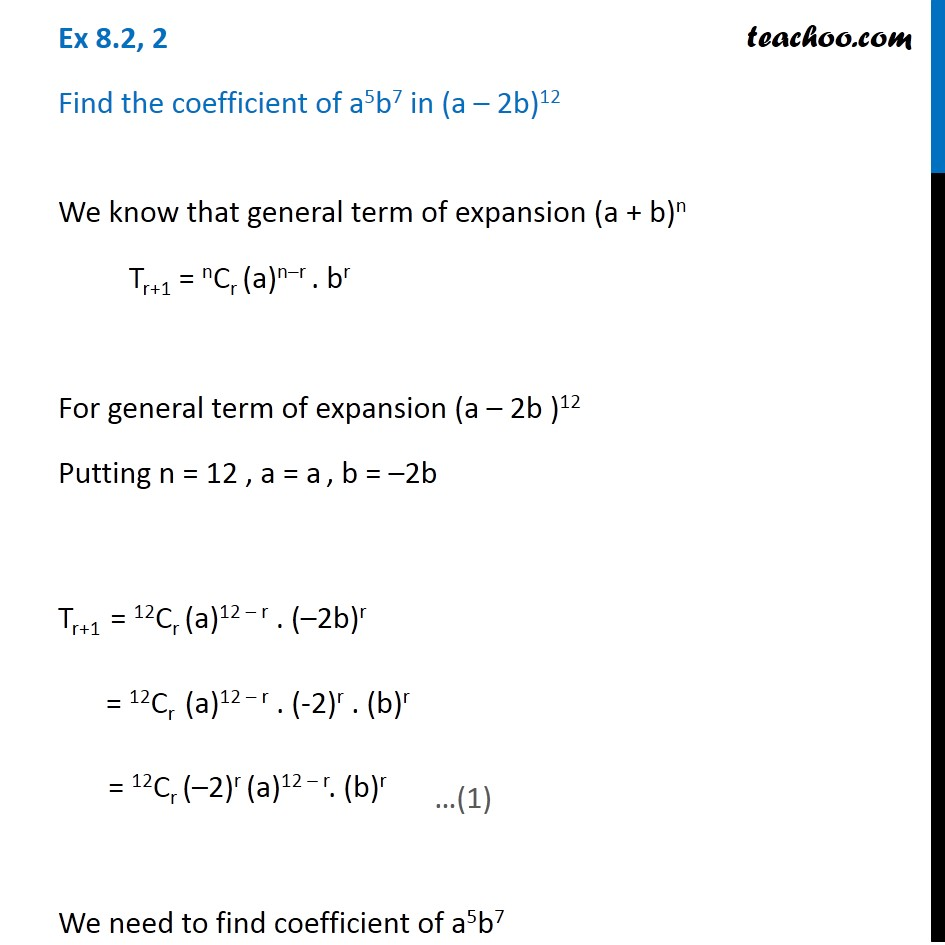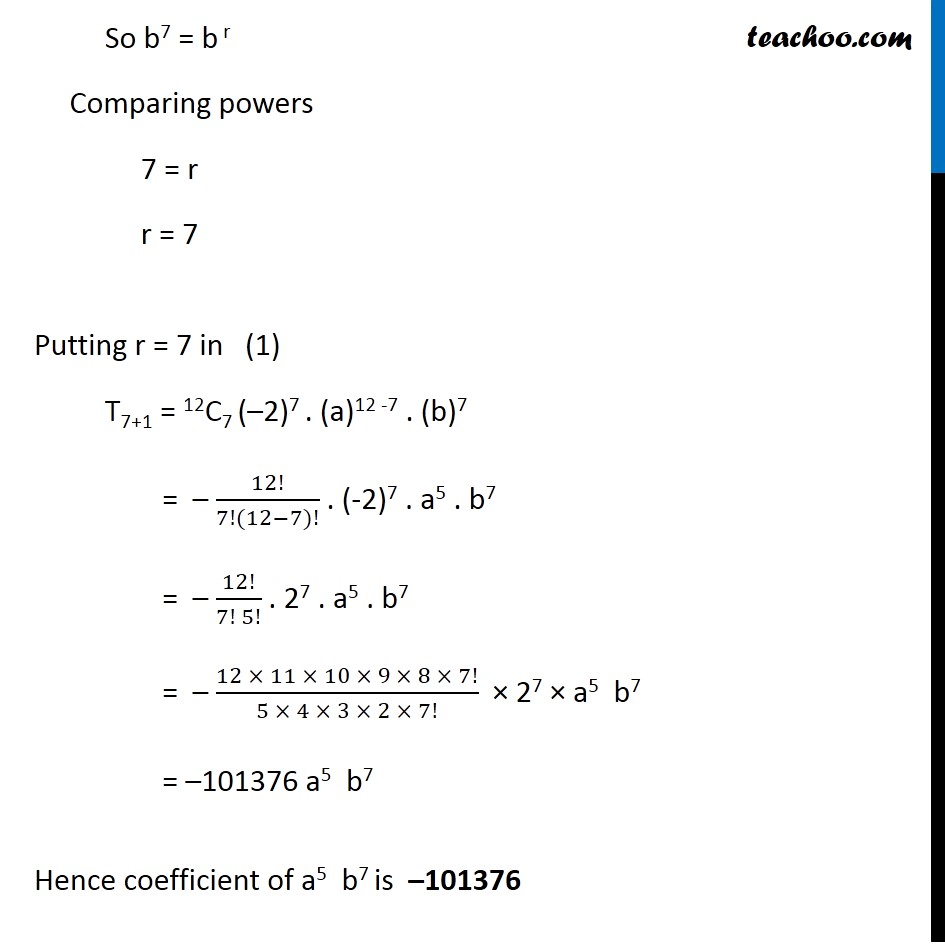General Term of Binomial Theorem

Chapter 7 Class 11 Binomial Theorem
Serial order wiseLearn in your speed, with individual attention - Teachoo Maths 1-on-1 Class

### Transcript

Question 2 Find the coefficient of a5b7 in (a – 2b)12 We know that general term of expansion (a + b)n Tr+1 = nCr (a)n–r . br For general term of expansion (a – 2b )12 Putting n = 12 , a = a , b = –2b Tr+1 = 12Cr (a)12 – r . (–2b)r = 12Cr (a)12 – r . (-2)r . (b)r = 12Cr (–2)r (a)12 – r. (b)r We need to find coefficient of a5b7 So b7 = b r Comparing powers 7 = r r = 7 Putting r = 7 in (1) T7+1 = 12C7 (–2)7 . (a)12 -7 . (b)7 = – 12!/(7!(12−7)!) . (-2)7 . a5 . b7 = – 12!/(7! 5!) . 27 . a5 . b7 = – (12 × 11 × 10 × 9 × 8 × 7!)/(5 × 4 × 3 × 2 × 7!) × 27 × a5 b7 = –101376 a5 b7 Hence coefficient of a5 b7 is –101376